Latest SSC jobs   »   Statement conclusion and missing number series...

# Statement conclusion and missing number series Questions of Reasoning for SSC CHSL 2020: 4th February 2020

Directions (1-2): A statement is given followed by two conclusions I and II. You have to consider the statement to be true, even if it seems to be at variance from commonly known facts. You are to decide which of the given conclusions can definitely be drawn from the given statement. Indicate your answer.

Q1. Statement :
Child rearing is an art; young parents needs training in child-rearing practices.
Conclusions :
I. Now-a-days young parents must be trained in child-rearing.
II. Training will enable the young couples to become better parents.

(a) Only I follows
(b) Only II follows
(c) Neither I nor II follows
(d) Both I and II follow

Q2. Statement :
Happiness derived from external materials is momentary. Everlasting happiness has to come from within.
Conclusions :
I. Nobody can experience happiness from outside
II. Happiness experienced from cinema is not lasting.

(a) Only I follows
(b) Only II follows
(c) Neither I nor II follow
(d) Both I and II follow

Directions (3-6): Select the missing number from the given responses.

Q3.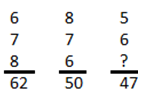(a) 4
(b) 7
(c) 8
(d) 9

Q4.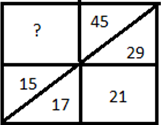(a) 8
(b) 10
(c) 14
(d) 16

Q5.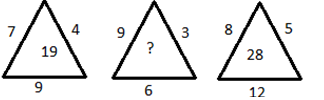(a) 27
(b) 21
(c) 28
(d) 17

Q6.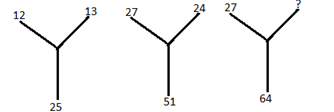(a) 35
(b) 36
(c) 37
(d) 38

Q7. select the missing number from the given responses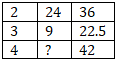(a) 10
(b) 16
(c) 12
(d) 32

Q8. select the missing number from the given responses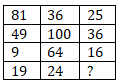(a) 25
(b) 15
(c) 77
(d) 67

Directions (9-10): some equations are solved on the basis of a certain system. Find the correct answer for the unsolved equation on that basis.

Q9.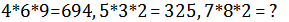(a) 729
(b) 872
(c) 827
(d) 27

Q10. If 2463 = 36 and 5552 = 30, then 6732 = ?
(a) 32
(b) 36
(c) 34
(d) 39

#### Solutions:

S1. Ans.(b)
Sol. Only II conclusion follows.

S2. Ans.(b)
Sol. Only II conclusion shows above statement.

S3. Ans.(b)
Sol. 7*8+6=62, 6*7+8=50 ,so ans is= (47-5)/6=7

S4. Ans.(c)
Sol. difference =1,2,4,8,16. So, 14 will be the ans.

S5. Ans.(b)
Sol. 7*4-9=19, 9*3-6=21, 8*5-12=28

S6. Ans.(c)
Sol. 12+13=25,27+24=51, ans is=64-27=37

S7. Ans. (c)
Sol.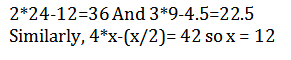S8. Ans. (b)
Sol. 9+7+3=19
6+10+8=24
Similarly , 5+6+4 = 15

S9. Ans. (c)
Sol. Jumbling of the digits

S10. Ans. (a)
Sol.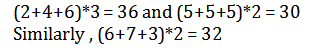### SSC CGL | SSC CHSL 2020 | Order & Ranking | Reasoning Marathon Class

You may also like to read:

#### Congratulations!General Awareness & Science Capsule PDF